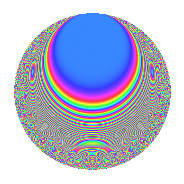# Properties

 Label 671.2.aLevel 671 Weight 2 Character orbit a Rep. character $$\chi_{671}(1,\cdot)$$ Character field $$\Q$$ Dimension 51 Newforms 4 Sturm bound 124 Trace bound 1

# Related objects

## Defining parameters

 Level: $$N$$ = $$671 = 11 \cdot 61$$ Weight: $$k$$ = $$2$$ Character orbit: $$[\chi]$$ = 671.a (trivial) Character field: $$\Q$$ Newforms: $$4$$ Sturm bound: $$124$$ Trace bound: $$1$$ Distinguishing $$T_p$$: $$2$$

## Dimensions

The following table gives the dimensions of various subspaces of $$M_{2}(\Gamma_0(671))$$.

Total New Old
Modular forms 64 51 13
Cusp forms 61 51 10
Eisenstein series 3 0 3

The following table gives the dimensions of the cuspidal new subspaces with specified eigenvalues for the Atkin-Lehner operators and the Fricke involution.

$$11$$$$61$$FrickeDim.
$$+$$$$+$$$$+$$$$6$$
$$+$$$$-$$$$-$$$$21$$
$$-$$$$+$$$$-$$$$19$$
$$-$$$$-$$$$+$$$$5$$
Plus space$$+$$$$11$$
Minus space$$-$$$$40$$

## Trace form

 $$51q$$ $$\mathstrut +\mathstrut 3q^{2}$$ $$\mathstrut +\mathstrut 2q^{3}$$ $$\mathstrut +\mathstrut 57q^{4}$$ $$\mathstrut +\mathstrut 4q^{5}$$ $$\mathstrut +\mathstrut 8q^{7}$$ $$\mathstrut -\mathstrut 9q^{8}$$ $$\mathstrut +\mathstrut 61q^{9}$$ $$\mathstrut +\mathstrut O(q^{10})$$ $$51q$$ $$\mathstrut +\mathstrut 3q^{2}$$ $$\mathstrut +\mathstrut 2q^{3}$$ $$\mathstrut +\mathstrut 57q^{4}$$ $$\mathstrut +\mathstrut 4q^{5}$$ $$\mathstrut +\mathstrut 8q^{7}$$ $$\mathstrut -\mathstrut 9q^{8}$$ $$\mathstrut +\mathstrut 61q^{9}$$ $$\mathstrut +\mathstrut 6q^{10}$$ $$\mathstrut -\mathstrut 3q^{11}$$ $$\mathstrut +\mathstrut 8q^{12}$$ $$\mathstrut +\mathstrut 14q^{13}$$ $$\mathstrut +\mathstrut 4q^{14}$$ $$\mathstrut +\mathstrut 2q^{15}$$ $$\mathstrut +\mathstrut 73q^{16}$$ $$\mathstrut +\mathstrut 2q^{17}$$ $$\mathstrut -\mathstrut q^{18}$$ $$\mathstrut +\mathstrut 16q^{19}$$ $$\mathstrut -\mathstrut 14q^{20}$$ $$\mathstrut +\mathstrut 20q^{21}$$ $$\mathstrut +\mathstrut 3q^{22}$$ $$\mathstrut -\mathstrut 2q^{23}$$ $$\mathstrut +\mathstrut 8q^{24}$$ $$\mathstrut +\mathstrut 67q^{25}$$ $$\mathstrut +\mathstrut 10q^{26}$$ $$\mathstrut -\mathstrut 34q^{27}$$ $$\mathstrut +\mathstrut 12q^{28}$$ $$\mathstrut +\mathstrut 10q^{29}$$ $$\mathstrut -\mathstrut 12q^{30}$$ $$\mathstrut +\mathstrut 6q^{31}$$ $$\mathstrut +\mathstrut 15q^{32}$$ $$\mathstrut -\mathstrut 2q^{33}$$ $$\mathstrut +\mathstrut 6q^{34}$$ $$\mathstrut -\mathstrut 28q^{35}$$ $$\mathstrut +\mathstrut 101q^{36}$$ $$\mathstrut +\mathstrut 16q^{37}$$ $$\mathstrut -\mathstrut 20q^{38}$$ $$\mathstrut -\mathstrut 8q^{39}$$ $$\mathstrut -\mathstrut 6q^{40}$$ $$\mathstrut +\mathstrut 10q^{41}$$ $$\mathstrut -\mathstrut 20q^{42}$$ $$\mathstrut +\mathstrut 36q^{43}$$ $$\mathstrut -\mathstrut 11q^{44}$$ $$\mathstrut +\mathstrut 22q^{45}$$ $$\mathstrut +\mathstrut 24q^{46}$$ $$\mathstrut -\mathstrut 12q^{47}$$ $$\mathstrut -\mathstrut 64q^{48}$$ $$\mathstrut +\mathstrut 91q^{49}$$ $$\mathstrut +\mathstrut 29q^{50}$$ $$\mathstrut +\mathstrut 32q^{51}$$ $$\mathstrut +\mathstrut 22q^{52}$$ $$\mathstrut +\mathstrut 6q^{53}$$ $$\mathstrut -\mathstrut 16q^{54}$$ $$\mathstrut -\mathstrut 8q^{55}$$ $$\mathstrut +\mathstrut 24q^{56}$$ $$\mathstrut +\mathstrut 44q^{57}$$ $$\mathstrut -\mathstrut 14q^{58}$$ $$\mathstrut -\mathstrut 34q^{59}$$ $$\mathstrut -\mathstrut 28q^{60}$$ $$\mathstrut +\mathstrut q^{61}$$ $$\mathstrut +\mathstrut 4q^{63}$$ $$\mathstrut +\mathstrut 113q^{64}$$ $$\mathstrut +\mathstrut 48q^{65}$$ $$\mathstrut -\mathstrut 8q^{66}$$ $$\mathstrut +\mathstrut 22q^{67}$$ $$\mathstrut -\mathstrut 18q^{68}$$ $$\mathstrut -\mathstrut 34q^{69}$$ $$\mathstrut -\mathstrut 28q^{70}$$ $$\mathstrut -\mathstrut 18q^{71}$$ $$\mathstrut -\mathstrut 37q^{72}$$ $$\mathstrut +\mathstrut 22q^{73}$$ $$\mathstrut -\mathstrut 26q^{74}$$ $$\mathstrut -\mathstrut 52q^{75}$$ $$\mathstrut +\mathstrut 4q^{76}$$ $$\mathstrut +\mathstrut 8q^{77}$$ $$\mathstrut -\mathstrut 112q^{78}$$ $$\mathstrut +\mathstrut 48q^{79}$$ $$\mathstrut -\mathstrut 50q^{80}$$ $$\mathstrut +\mathstrut 123q^{81}$$ $$\mathstrut -\mathstrut 42q^{82}$$ $$\mathstrut -\mathstrut 4q^{83}$$ $$\mathstrut -\mathstrut 60q^{84}$$ $$\mathstrut +\mathstrut 56q^{85}$$ $$\mathstrut -\mathstrut 32q^{86}$$ $$\mathstrut -\mathstrut 36q^{87}$$ $$\mathstrut +\mathstrut 15q^{88}$$ $$\mathstrut -\mathstrut 126q^{90}$$ $$\mathstrut +\mathstrut 48q^{91}$$ $$\mathstrut +\mathstrut 12q^{92}$$ $$\mathstrut -\mathstrut 42q^{93}$$ $$\mathstrut +\mathstrut 12q^{94}$$ $$\mathstrut -\mathstrut 36q^{95}$$ $$\mathstrut -\mathstrut 44q^{96}$$ $$\mathstrut +\mathstrut 60q^{97}$$ $$\mathstrut -\mathstrut 77q^{98}$$ $$\mathstrut -\mathstrut 9q^{99}$$ $$\mathstrut +\mathstrut O(q^{100})$$

## Decomposition of $$S_{2}^{\mathrm{new}}(\Gamma_0(671))$$ into irreducible Hecke orbits

Label Dim. $$A$$ Field CM Traces A-L signs $q$-expansion
$$a_2$$ $$a_3$$ $$a_5$$ $$a_7$$ 11 61
671.2.a.a $$5$$ $$5.358$$ 5.5.24217.1 None $$-2$$ $$0$$ $$-2$$ $$-1$$ $$-$$ $$-$$ $$q+\beta _{1}q^{2}+(-\beta _{1}+\beta _{4})q^{3}+(-2\beta _{1}+\cdots)q^{4}+\cdots$$
671.2.a.b $$6$$ $$5.358$$ 6.6.2661761.1 None $$0$$ $$-1$$ $$-1$$ $$-5$$ $$+$$ $$+$$ $$q+\beta _{3}q^{2}-\beta _{1}q^{3}-\beta _{5}q^{4}+(\beta _{1}-\beta _{3}+\cdots)q^{5}+\cdots$$
671.2.a.c $$19$$ $$5.358$$ $$\mathbb{Q}[x]/(x^{19} - \cdots)$$ None $$5$$ $$0$$ $$0$$ $$9$$ $$-$$ $$+$$ $$q+\beta _{1}q^{2}+\beta _{11}q^{3}+(1+\beta _{2})q^{4}-\beta _{3}q^{5}+\cdots$$
671.2.a.d $$21$$ $$5.358$$ None $$0$$ $$3$$ $$7$$ $$5$$ $$+$$ $$-$$

## Decomposition of $$S_{2}^{\mathrm{old}}(\Gamma_0(671))$$ into lower level spaces

$$S_{2}^{\mathrm{old}}(\Gamma_0(671)) \cong$$ $$S_{2}^{\mathrm{new}}(\Gamma_0(11))$$$$^{\oplus 2}$$$$\oplus$$$$S_{2}^{\mathrm{new}}(\Gamma_0(61))$$$$^{\oplus 2}$$